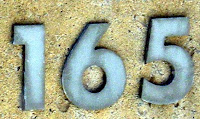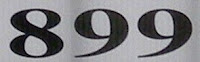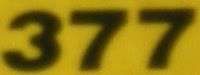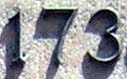## Thursday, December 23, 2010

### 2011

2011 is a prime number.

2011 has the representation 211 - 37.

2011 is the sum of 11 consecutive primes: 2011 = 157 + 163 + 167 + 173 + 179 + 181 + 191 + 193 + 197 + 199 + 211.

2011 is a number such that first reversing the digits and then squaring equals the result of first squaring and then reversing the digits: 11022 =1214404 and 20112 = 4044121.

28 is 2011 in base 5.

The sum of the digits of 2011 is equal to the number of digits: 2 + 0 + 1 + 1 = 4. The digit sum is a square and a power of 2.The next prime date from now (month, day, and year are primes) is 2/2/2011. Note that 222011 is a prime.

In the Aeon Flux television series and film (2005), 99 percent of the world's population is wiped out by a mysterious virus in the year 2011.

The Peace Corps marks its 50th anniversary in the year 2011. It is also the 5oth anniversary of the first manned mission to space.

2011 is the 100th anniversary of the launch of the Titanic on May 31, 1911.

2011 is designated the International Year of Chemistry.## Wednesday, December 22, 2010

### 982

982 = 2 x 491.

982 is the number of integer partitions of 39 into distinct parts.

982 is the number of ways to place four non-attacking queens on a 7 x 7 board.

Written backwards, 982 is the square 289.NGC 982 is a spiral galaxy in the constellation Andromeda.

## Tuesday, December 21, 2010

### 165

165 = 3 x 5 x 11.

165 is 10100101 in base 2 (binary).

165 is the number of integer partitions of 26 into distinct parts.

165 is the ninth tetrahedral number.

165 is the sum of the divisors of the first 14 integers.The Tahoe Rim Trail is a 165-mile hiking trail around Lake Tahoe.

Source: WolframAlpha

## Monday, December 20, 2010

### 899

899 = 29 x 31. It is the product of two successive primes and the product of twin primes.

Any number consisting of an 8 followed by an even number of nines has prime factors 3 x (10n/2) + 1 and 3 x (10n/2) - 1, where n is the number of nines: 899 = 3(101) + 1) x 3(101) - 1 = 31 x 29. Similarly, 8999999 = 2999 x 3001.

899 is the smallest number whose sum of digits is 26.

899 is a number that can't be expressed as the sum of a prime number and a Fibonacci number.The Benneli TNT 899 Sport is an Italian motorcycle.

## Friday, December 17, 2010

### 377

377 = 13 x 29.

377 has two representations as a sum of two squares: 377 = 42 + 192 = 112 + 162.

377 is the fourteenth Fibonacci number. It is the largest known Fibonacci number whose digits are all prime.

377 is the hypotenuse of two primitive Pythagorean triples: 3772 = 1352 + 3522 = 1522 + 3452.

377 is the sum of the squares of the first six primes: 377 = 22 + 32 + 52 + 72 + 112 + 132.The Aero Spacelines B-377 SG Super Guppy is a four-engine oversize-cargo freighter aircraft.

## Thursday, December 16, 2010

### 1196

1196 = 22 x 13 x 23.

1196 is the one thousandth non-prime number.

1196 is the number of lines through exactly two points of a 9 x 9 grid of points.

1196 is 14241 in base 5 and 616 in base 14.

1196 is the number of assymetric polyominoes made up of 9 cells.NGC 1196 is a galaxy in the constellation Eridanus, discovered by John Herschel in 1835.

## Wednesday, December 15, 2010

### 359

359 is a prime number. It is a Sophie Germain prime because 2 x 359 + 1 = 719, a prime.

359 is a number that cannot be written as a sum of three squares.

359 = -1 + 23 x 45.

The 359th, 360th, and 361st decimal digits of pi are 360.

359 has a base 3 representation (111022) that ends with its base 7 representation (1022), which is also true for 357 and 358.In the Star Trek imaginary universe, the Battle of Wolf 359 was the Federation's first major battle against a group of cyborgs.

## Tuesday, December 14, 2010

### 173

173 is a prime number. It is a Sophie Germain prime because 2 x 173 + 1 = 347, which is also prime.

173 has a representation as a sum of two squares: 173 = 22 + 132. It is the sum of the squares of two Fibonacci numbers, 2 and 13.

173 is the hypotenuse of a primitive Pythagorean triple: 1732 = 522 + 1652.

173 is the only prime whose sum of cubed digits equals its reversal: 13 + 73 + 33 = 371.

173 is the largest known prime whose square (29929) and cube (5177717) have no digits in common.

173 = 1 + 22 + 23 + 25 + 27, where the exponents are consecutive primes.In the 1993 movie The Sandlot, The Beast (a ferocious dog) is said to have eaten 173 guys.

## Monday, December 13, 2010

### 21

21 = 3 x 7.

21 is 10101 in base 2 (binary) and 111 in base 4.

21 has a unique representation as a sum of three squares: 21 = 12 + 22 + 42.

21 is the smallest number with five representations as a sum of three primes: 21 = 2 + 2 + 17 = 3 + 5 + 13 = 3 + 7 + 11 = 5 + 5 + 11 = 7 + 7 + 7.

21 is the eighth Fibonacci number.

21 is the fifth Motzkin number.

21 is the sixth triangular number.21 is the total number of spots on a normal die.

Source: Phillips, R. 2004. Numbers: Facts, Figures and Fiction. Badsey Publications.

## Friday, December 10, 2010

### 364

364 = 22 x 7 x 13.

364 has a unique representation as a sum of three squares: 364 = 22 + 62 + 182.

364 is the twelfth tetrahedral number.

364 is 111111 in base 3.

The first two digits of 364 are the product of the first two primes to the power of the first prime and the last two digits are the first prime to the product of the first two primes: 36 = (2 x 3)2 and 64 = 2(2 x 3).Asteroid 364 Isara was discovered in 1893.

## Thursday, December 9, 2010

### 954

954 = 2 x 32 x 53.

954 has a representation as a sum of two squares: 954 = 152 + 272.

954 is the smallest number n such that if you subtract its reverse from it, you get a number with the same digits as n: 954 - 459 = 495.

954 is 676 in base 12 and 585 in base 13.Steak 954 is a steakhouse in Fort Lauderdale, Florida.

## Wednesday, December 8, 2010

### 461

461 is a prime number.

461 has a representation as a sum of two squares: 461 = 102 + 192.

461 and 463 form a twin prime pair.

461 is the hypotenuse of a primitive Pythagorean triple: 4612 = 2612 + 3802.

461 is the largest number for which ((102n) + 1)/101 is known to be a prime number.Eric Clapton recorded a solo album titled 461 Ocean Boulevard.

## Tuesday, December 7, 2010

### 565

565 = 5 x 113.

565 has two representations as a sum of two squares: 565 = 62 + 232 = 92 + 222.

565 is the hypotenuse of two primitive Pythagorean triples: 5652 = 2762 + 4932 = 3962 + 4032.

565 is 474 in base 11.

565 is the sum of three consecutive primes: 565 = 181 + 191 + 193.in the year 565, St. Columba reported seeing a monster in Loch Ness, Scotland.

Source: WolframAlpha

## Monday, December 6, 2010

### 942

942 = 2 x 3 x 157.

942 is 666 in base 12.

942 is the smallest number whose cube contains five 8s: 9423 = 835,896,888.

942 is the sum of four consecutive primes: 942 = 229 + 233 + 239 + 241.NGC 942/943 (also known as Arp 309) is a pair of interacting galaxies in the constellation Taurus.

## Friday, December 3, 2010

### 877

877 is a prime number.

877 has a representation as a sum of two squares: 877 = 62 + 292.

877 is the seventh Bell number.

877 is the hypotenuse of a primitive Pythagorean triple: 8772 = 3482 + 8052.

877 is 1555 in base 8 and 611 in base 12.877 is a telephone area code for toll-free service.

## Thursday, December 2, 2010

### 451

451 = 11 x41.

451 is a decagonal number. It is also a centered decagonal number.

10451 + 451 is a prime number.

3 plus the integer formed by the first 451 digits after the decimal point in pi is a prime number.The title of the novel and film Fahrenheit 451 refers to the temperature at which book paper supposedly burns.

## Wednesday, December 1, 2010

### 933

933 = 3 x 311.

933 is a number that can't be expressed as a sum of a prime number and a Fibonacci number.

933 is a house number.

933 is a member of the sequence generated when you start with 1 and repeatedly reverse the digits and add 10 to get the next term: 1, 11, 21, 32, 33, . . . , 329, 933, 349, . . .Asteroid 933 Susi was discovered in 1927.